### A.32 The evo­lu­tion of prob­a­bil­ity

This note looks at con­ser­va­tion of prob­a­bil­ity, and the re­sult­ing de­f­i­n­i­tions of the re­flec­tion and trans­mis­sion co­ef­fi­cients in scat­ter­ing. It also ex­plains the con­cept of the “prob­a­bil­ity cur­rent” that you may oc­ca­sion­ally run into.

For the un­steady Schrö­din­ger equa­tion to pro­vide a phys­i­cally cor­rect de­scrip­tion of non­rel­a­tivis­tic quan­tum me­chan­ics, par­ti­cles should not be able to dis­ap­pear into thin air. In par­tic­u­lar, dur­ing the evo­lu­tion of the wave func­tion of a sin­gle par­ti­cle, the to­tal prob­a­bil­ity of find­ing the par­ti­cle if you look every­where should stay one at all times: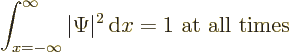For­tu­nately, the Schrö­din­ger equa­tion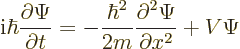does in­deed con­serve this to­tal prob­a­bil­ity, so all is well.

To ver­ify this, note first that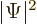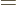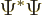, where the star in­di­cates the com­plex con­ju­gate, so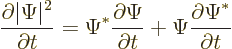To get an ex­pres­sion for that, take the Schrö­din­ger equa­tion above times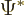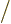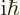and add the com­plex con­ju­gate of the Schrö­din­ger equa­tion,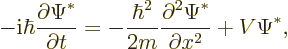times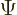. The po­ten­tial en­ergy terms drop out, and what is left is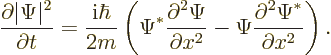Now it can be ver­i­fied by dif­fer­en­ti­at­ing out that the right hand side can be rewrit­ten as a de­riv­a­tive: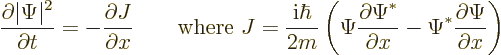(A.232)

For rea­sons that will be­come ev­i­dent be­low,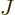is called the “prob­a­bil­ity cur­rent.” Note that, like, will be zero at in­fi­nite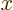for proper, nor­mal­ized wave func­tions.

If (A.232) is in­te­grated over all, the de­sired re­sult is ob­tained: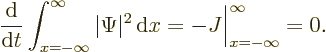There­fore, the to­tal prob­a­bil­ity of find­ing the par­ti­cle does not change with time. If a proper ini­tial con­di­tion is pro­vided to the Schrö­din­ger equa­tion in which the to­tal prob­a­bil­ity of find­ing the par­ti­cle is one, then it stays one for all time.

It gets a lit­tle more in­ter­est­ing to see what hap­pens to the prob­a­bil­ity of find­ing the par­ti­cle in some given fi­nite re­gion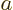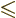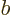. That prob­a­bil­ity is given by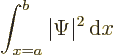and it can change with time. A wave packet might en­ter or leave the re­gion. In par­tic­u­lar, in­te­gra­tion of (A.232) gives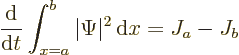This can be un­der­stood as fol­lows: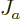is the prob­a­bil­ity flow­ing out of the re­gion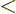into the in­ter­val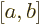through the end. That in­creases the prob­a­bil­ity within. Sim­i­larly,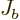is the prob­a­bil­ity flow­ing out ofatinto the re­gion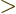; it de­creases the prob­a­bil­ity within. Now you see whyis called prob­a­bil­ity cur­rent; it is equiv­a­lent to a stream of prob­a­bil­ity in the pos­i­tive-​di­rec­tion.

The prob­a­bil­ity cur­rent can be gen­er­al­ized to more di­men­sions us­ing vec­tor cal­cu­lus: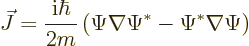(A.233)

and the net prob­a­bil­ity flow­ing out of a re­gion is given by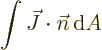(A.234)

where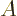is the out­side sur­face area of the re­gion, and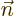is a unit vec­tor nor­mal to the sur­face. A sur­face in­te­gral like this can of­ten be sim­pli­fied us­ing the di­ver­gence (Gauss or what­ever) the­o­rem of cal­cu­lus.

Re­turn­ing to the one-di­men­sion­al case, it is of­ten de­sir­able to re­late con­ser­va­tion of prob­a­bil­ity to the en­ergy eigen­func­tions of the Hamil­ton­ian,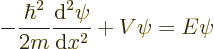be­cause the en­ergy eigen­func­tions are generic, not spe­cific to one par­tic­u­lar ex­am­ple wave func­tion.

To do so, first an im­por­tant quan­tity called the “Wron­skian” must be in­tro­duced. Con­sider any two eigen­func­tions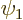and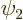of the Hamil­ton­ian: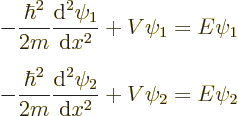If you mul­ti­ply the first equa­tion above by, the sec­ond byand then sub­tract the two, you get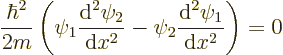The con­stant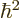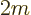can be di­vided out, and by dif­fer­en­ti­a­tion it can be ver­i­fied that the re­main­der can be writ­ten as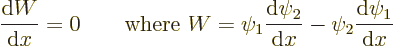The quan­tity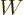is called the Wron­skian. It is the same at all val­ues of.

As an ap­pli­ca­tion, con­sider the ex­am­ple po­ten­tial of fig­ure A.11 in ad­den­dum {A.27} that bounces a par­ti­cle com­ing in from the far left back to where it came from. In the left re­gion, the po­ten­tialhas a con­stant value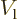. In this re­gion, an en­ergy eigen­func­tion is of the form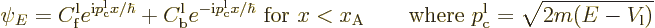At the far right, the po­ten­tial grows with­out bound and the eigen­func­tion be­comes zero rapidly. To make use of the Wron­skian, take the first so­lu­tionto be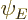it­self, andto be its com­plex con­ju­gate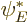. Since at the far right the eigen­func­tion be­comes zero rapidly, the Wron­skian is zero there. And since the Wron­skian is con­stant, that means it must be zero every­where. Next, if you plug the above ex­pres­sion for the eigen­func­tion in the left re­gion into the de­f­i­n­i­tion of the Wron­skian and clean up, you get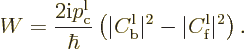If that is zero, the mag­ni­tude of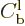must be the same as that of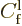.

This can be un­der­stood as fol­lows: if a wave packet is cre­ated from eigen­func­tions with ap­prox­i­mately the same en­ergy, then the terms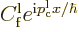com­bine for large neg­a­tive times into a wave packet com­ing in from the far left. The prob­a­bil­ity of find­ing the par­ti­cle in that wave packet is pro­por­tional to the in­te­grated square mag­ni­tude of the wave func­tion, hence pro­por­tional to the square mag­ni­tude of. For large pos­i­tive times, the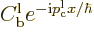terms com­bine in a sim­i­lar wave packet, but one that re­turns to­wards the far left. The prob­a­bil­ity of find­ing the par­ti­cle in that de­part­ing wave packet must still be the same as that for the in­com­ing packet, so the square mag­ni­tude ofmust be the same as that of.

Next con­sider a generic scat­ter­ing po­ten­tial like the one in fig­ure 7.22. To the far left, the eigen­func­tion is again of the form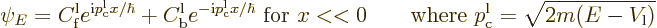while at the far right it is now of the form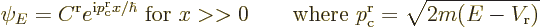The Wron­skian can be found the same way as be­fore: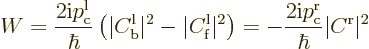The frac­tion of the in­com­ing wave packet that ends up be­ing re­flected back to­wards the far left is called the “re­flec­tion co­ef­fi­cient”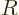. Fol­low­ing the same rea­son­ing as above, it can be com­puted from the co­ef­fi­cients in the far left re­gion of con­stant po­ten­tial as: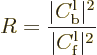The re­flec­tion co­ef­fi­cient gives the prob­a­bil­ity that the par­ti­cle can be found to the left of the scat­ter­ing re­gion at large times.

Sim­i­larly, the frac­tion of the in­com­ing wave packet that passes through the po­ten­tial bar­rier to­wards the far right is called the “trans­mis­sion co­ef­fi­cient”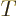. It gives the prob­a­bil­ity that the par­ti­cle can be found to the right of the scat­ter­ing re­gion at large times. Be­cause of con­ser­va­tion of prob­a­bil­ity,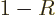.

Al­ter­na­tively, be­cause of the Wron­skian ex­pres­sion above, the trans­mis­sion co­ef­fi­cient can be ex­plic­itly com­puted from the co­ef­fi­cient of the eigen­func­tion in the far right re­gion as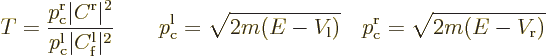If the po­ten­tial en­ergy is the same at the far right and far left, the two clas­si­cal mo­menta are the same,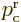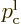. Oth­er­wise, the rea­son that the ra­tio of clas­si­cal mo­menta ap­pears in the trans­mis­sion co­ef­fi­cient is be­cause the clas­si­cal mo­menta in a wave packet have a dif­fer­ent spac­ing with re­spect to en­ergy if the po­ten­tial en­ergy is dif­fer­ent. (The above ex­pres­sion for the trans­mis­sion co­ef­fi­cient can also be de­rived ex­plic­itly us­ing the Par­se­val equal­ity of Fourier analy­sis, in­stead of in­ferred from con­ser­va­tion of prob­a­bil­ity and the con­stant Wron­skian.)